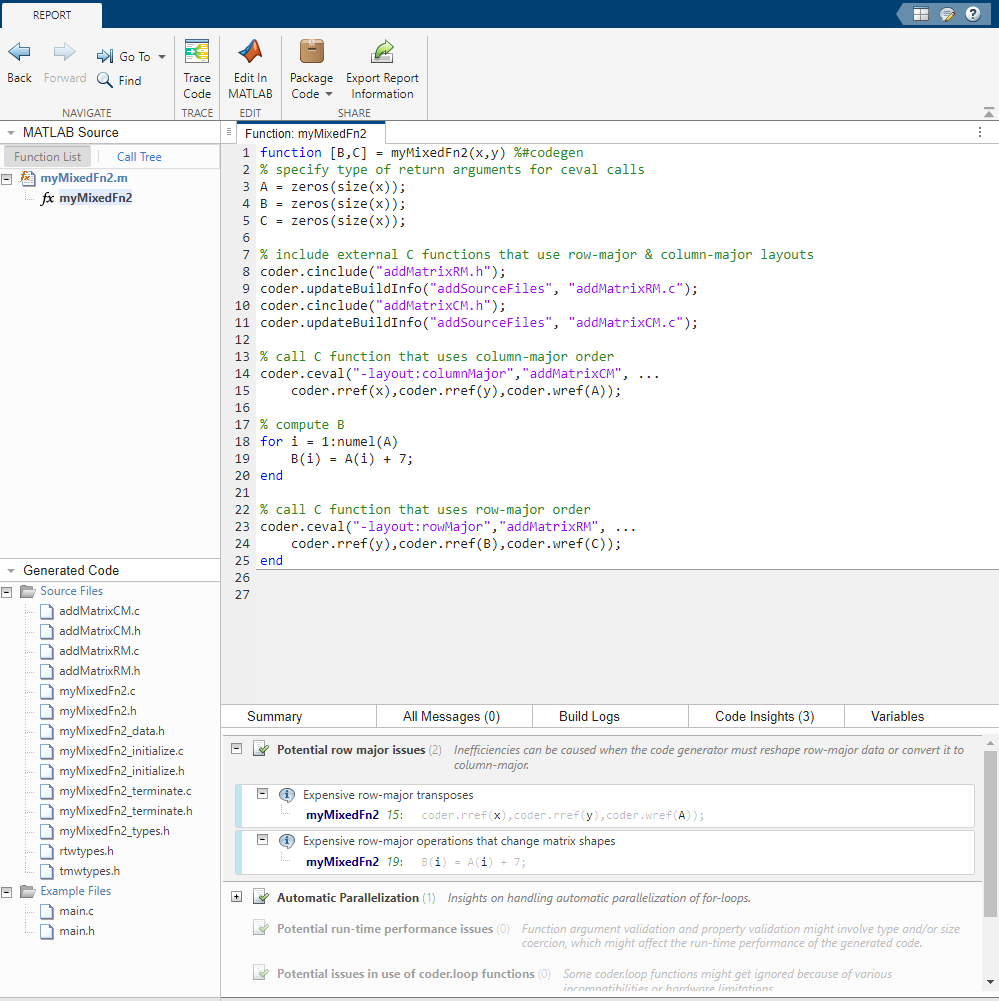## Code Design for Row-Major Array Layout

Outside of code generation, MATLAB® uses column-major layout by default. Array layout specifications do not affect self-contained MATLAB code. To test the efficiency of your generated code or your MATLAB Function block, create separate versions with row-major layout and column-major layout. Then, compare their performance.

You can design your MATLAB code to avoid potential inefficiencies related to array layout. Inefficiencies can be caused by:

• Conversions between row-major layout and column-major layout.

• One-dimensional or linear indexing of row-major data.

• Reshaping or rearrangement of row-major data.

Array layout conversions are necessary when you mix row-major and column-major specifications in the same code or model, or when you use linear indexing on data that is stored in row-major. When you simulate a model or generate code for a model that uses column-major, and that contains a MATLAB Function block that uses row-major, then the software converts input data to row-major and output data back to column-major as needed, and vice versa.

Inefficiencies can be caused by functions or algorithms that are less optimized for a given choice of array layout. If a function or algorithm is more efficient for a different layout, you can enforce that layout by embedding it in another function with a `coder.rowMajor` or `coder.columnMajor` call.

### Understand Potential Inefficiencies Caused by Array Layout

Consider the code for `myMixedFn2`, which uses `coder.ceval` to pass data with row-major and column-major layout:

```function [B, C] = myMixedFn2(x,y) %#codegen % specify type of return arguments for ceval calls A = zeros(size(x)); B = zeros(size(x)); C = zeros(size(x)); % include external C functions that use row-major & column-major coder.cinclude('addMatrixRM.h'); coder.updateBuildInfo('addSourceFiles', 'addMatrixRM.c'); coder.cinclude('addMatrixCM.h'); coder.updateBuildInfo('addSourceFiles', 'addMatrixCM.c'); % call C function that uses column-major order coder.ceval('-layout:columnMajor','addMatrixCM', ... coder.rref(x),coder.rref(y),coder.wref(A)); % compute B for i = 1:numel(A) B(i) = A(i) + 7; end % call C function that uses row-major order coder.ceval('-layout:rowMajor','addMatrixRM', ... coder.rref(y),coder.rref(B),coder.wref(C)); end```

The external files are:

Declare the configuration object, `cfg`. Generate code that uses row-major layout by using the `-rowmajor` option.

```cfg = coder.config('lib'); cfg.HighlightPotentialRowMajorIssues = true; codegen myMixedFn2 -args {ones(20,10),ones(20,10)} -config cfg -launchreport -rowmajor ```

Highlighted issues are displayed in the code generation report, on the Code Insights tab, under the Potential row major issues section.Array layout inefficiencies occur here because:

• The code generator must convert the input variables `x` and `y` to column-major layout before passing them to `addMatrixCM`. Transposes must be inserted into the generated code.

• The code generator must transpose the output variable `A` back into row-major layout, because `myMixedFn2` uses row-major layout.

• The for-loop uses linear indexing, which requires column-major data. The code generator must recalculate the linear indexing because variables `A` and `B` are stored in row-major.

### Linear Indexing Uses Column-Major Array Layout

The code generator follows MATLAB column-major semantics for linear indexing. For more information on linear indexing in MATLAB, see Array Indexing (MATLAB).

To use linear indexing on row-major data, the code generator must first recalculate the data representation in column-major layout. This additional processing can slow performance. To improve code efficiency, avoid using linear indexing on row-major data, or use column-major layout for code that uses linear indexing.

For example, consider the function `sumShiftedProducts`, which accepts a matrix as an input and outputs a scalar value. The function uses linear indexing on the input matrix to sum up the product of each matrix element with an adjacent element. The output value of this operation depends on the order in which the input elements are stored.

```function mySum = sumShiftedProducts(A) %#codegen mySum = 0; % create linear vector of A elements B = A(:); % multiply B by B with elements shifted by one, and take sum mySum = sum( B.*circshift(B,1) ); end ```

For MATLAB Coder™, to generate code that uses row-major layout, enter:

`codegen -config:mex sumShiftedProducts -args {ones(2,3)} -launchreport -rowmajor`

For an example input, consider the matrix:

`D = reshape(1:6,3,2)'`

which yields:

```D = 1 2 3 4 5 6 ```

If you pass this matrix as input to the generated code, the elements of `A` are stored in the order:

` 1 2 3 4 5 6`

In contrast, because the vector `B` is obtained by linear indexing, it is stored in the order:

` 1 4 2 5 3 6`

The code generator must insert a reshaping operation to rearrange the data from row-major layout for `A` to column-major layout for `B`. This additional operation reduces the efficiency of the function for row-major layout. The inefficiency increases with the size of the array. Because linear indexing always uses column-major layout, the generated code for `sumShiftedProducts` produces the same output result whether generated with row-major layout or column-major layout.

In general, functions that compute indices or subscripts also use linear indexing, and produce results corresponding to data stored in column-major layout. These functions include: